QUESTION #413

# If I am standing on a mountain, Am I going faster than someone at sea level? If so, is my day shorter or will the higher vantage point of the horizon allow my day to seem longer?

The further away you are from the axis connecting the North and South Poles, the faster you will be moving as a result of Earth's rotation. So unless you are at either pole, climbing to a mountain top WILL cause you to move faster than at the same latitude at sea level. Your speed alone, however, has no effect on the relative length of night and day. One rotation still takes 24 hours...you are travelling faster because your path around the Earth is longer.

At higher altitudes you will see more sunlight (and more daylight time), though, because the higher vantage point allows you to see the Sun 'over the horizon' more than at sea level. Imagine being many thousands of miles above sea level, where the apparently smaller Earth is able to block out even less sunlight. At an infinite 'altitude', in interplanetary space, daylight is continuous.
Answered by: Paul Walorski, B.A., Part-time Physics Instructor

Yes, you will have longer daylight. For an observer exactly at sea level, the sun comes up at the horizon, which is exactly 90° away from straight up. Their 'sky' covers 180° of overhead angle with respect to their East-West line.' For an observer on a mountain, however, the curvature of the Earth allows for a larger 'sky.'' Their sun doesn't set until it has gone 90° plus some angle θ (see below).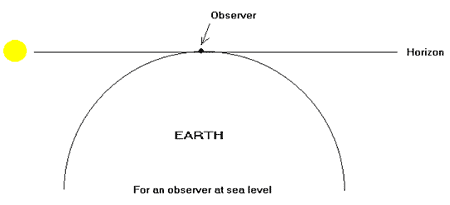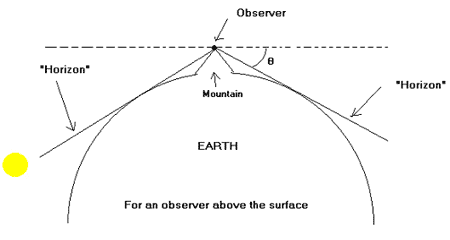The object of this derivation will be to calculate the angle θ as a function of R, the radius of the planet, and d, the distance above the surface of the planet.' We will then assume that the sun takes the same amount of time to travel through both observers' 180° sky, plus an extra 240 seconds per degree of extra angle for the observer on the mountain.

First of all, note that the 'horizon' looks a lot like one of those tangent line problems they make you do in calculus. This is where I will start. I will treat the mean surface of the Earth as a circle of radius R centered at the origin, and the observer as a point sitting at (0, R+d).' Our 'horizon' is a line that includes the observer point and is tangent to the circle at a point (x,y).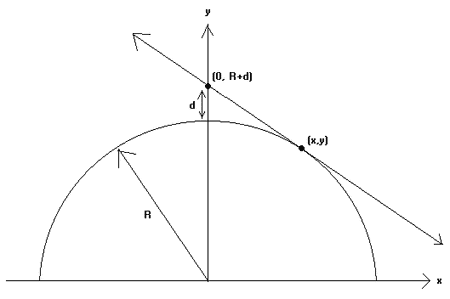First I want to know the values of x and y. I will start with the equation for a circle, since this point is on the circle.

1)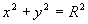Now, to find the slope of the line and the circle at that point, I will use implicit differentiation:

2)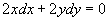3)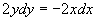4)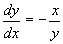I also know that the slope of the line at that point must be:

5)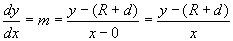Setting 5) equal to 4) (since the slope of the line equals the slope of the circle at the point in question), we get

6)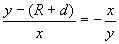And cross multiplying we get

7)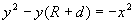or

8)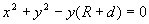Substituting equation 1) into the left side, we get:

9)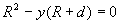10)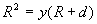11)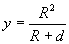Now to solve for our x-coordinate, which is a bit messy:

12)13)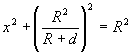14)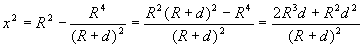Note to self:'suppress R2D2 joke''.D'oh!

15)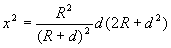16)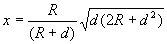Okay, now we have the coordinates of that point, and we can find the angle.' Using the following sketch, we see that

17)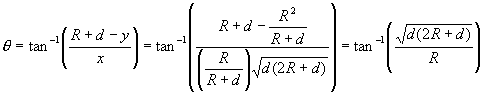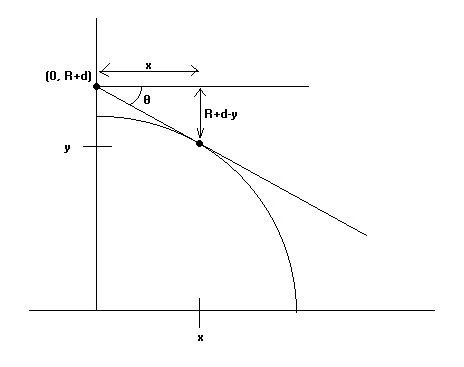Finally for the fun part, let's plug some numbers in. First note that if d=0, the extra angle is also zero, which is exactly what we'd expect.'Also, we see that

18)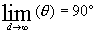Just as we'd expect. Using the radius of the Earth: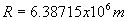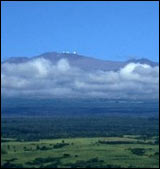and mountain height: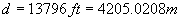which is the height of the Mauna Kea, one of five volcanic masses making up the 'Big Island' of Hawaii, (note that 13,796 ft or 4,205 m is the its part above the sea level - otherwise, from its base underneath the ocean to the top it is about 30,000 ft or 9,000 m tall, making it the tallest in the world when measured as such). I calculate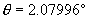which translates into 8.32 minutes of extra time for viewing the sunset.

Using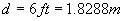you can see that a 6 foot tall person standing up has an extra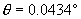over a man who's eyes are at sea level, which translates into approximately 10 seconds of sunset.' Of course, this small an angle could easily be obstructed by small things on the surface of the Earth, e.g. waves in the ocean.